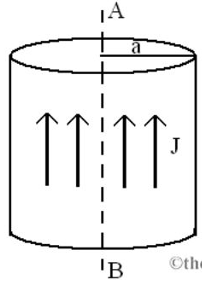# Problem: An electric current is flowing through a long cylindrical conductor with radius a = 0.15 m. The current density J= 5.5 A/m2 uniform in the cylinder. In this problem, we consider an imaginary cylinder with radius r around the axis AB (a) When r is greater than a, express the current inside the imaginary cylinder in terms of r, a, and J.(b) Express the magnitude of the magnetic field B at r &gt; a in terms i and r.

###### FREE Expert Solution

The electric current in a conductor:

Ampere's law:

(a)

At r greater than a, the current in the conductor is:

90% (442 ratings)###### Problem Details

An electric current is flowing through a long cylindrical conductor with radius a = 0.15 m. The current density J= 5.5 A/m2 uniform in the cylinder. In this problem, we consider an imaginary cylinder with radius r around the axis AB

(a) When r is greater than a, express the current inside the imaginary cylinder in terms of r, a, and J.

(b) Express the magnitude of the magnetic field B at r > a in terms i and r.